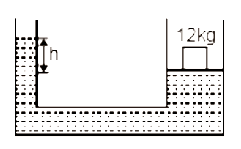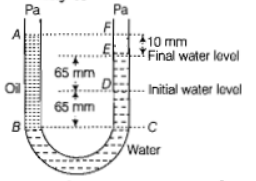#3 | Pascal's Law
(Physics) > Mechanical Properties of Fluids
Unable to watch the video, please try another server

#### My Notes

Create your notes while watching video by clicking on icon in video player.
Related Practice Questions :

The area of cross-section of the wider tube shown in the figure is  If a mass of 12 kg is placed on the massless piston, the difference is heights h in the level of water in the two tubes is :1.  10 cm

2.  6 cm

3.  15 cm

4.  2 cm

High Yielding Test Series + Question Bank - NEET 2020

Difficulty Level:

A U tube with both ends open to the atmosphere,is partially filled with water. Oil, which is immiscible with water, is poured into one side until it stands at a distance of 10mm above the water level on the other side. Meanwhile the water rises by 65 mm from its original level (see diagram). The density of the oil is(a)

(b)

(c)

(d)

High Yielding Test Series + Question Bank - NEET 2020

Difficulty Level:

Equal masses of water and a liquid of relative density 2 are mixed together, then the mixture has a density of
(a) 2/3                                 (b) 4/3
(c) 3/2                                  (d) 3

High Yielding Test Series + Question Bank - NEET 2020

Difficulty Level:

If two liquids of same masses but densities ${\mathrm{\rho }}_{1}$ and ${\mathrm{\rho }}_{2}$ respectively are mixed, then density of mixture is given by

(a) $\mathrm{\rho }=\frac{{\mathrm{\rho }}_{1}+{\mathrm{\rho }}_{2}}{2}$                             (b) $\mathrm{\rho }=\frac{{\mathrm{\rho }}_{1}+{\mathrm{\rho }}_{2}}{2{\mathrm{\rho }}_{1}{\mathrm{\rho }}_{2}}$

(c) $\mathrm{\rho }=\frac{2{\mathrm{\rho }}_{1}{\mathrm{\rho }}_{2}}{{\mathrm{\rho }}_{1}+{\mathrm{\rho }}_{2}}$                             (d) $\mathrm{\rho }=\frac{{\mathrm{\rho }}_{1}{\mathrm{\rho }}_{2}}{{\mathrm{\rho }}_{1}+{\mathrm{\rho }}_{2}}$

High Yielding Test Series + Question Bank - NEET 2020

Difficulty Level:

If two liquids of same volume but different densities ${\mathrm{\rho }}_{1}$ and ${\mathrm{\rho }}_{2}$ are mixed, then density of mixture is given by

(a) $\mathrm{\rho }=\frac{{\mathrm{\rho }}_{1}+{\mathrm{\rho }}_{2}}{2}$                          (b) $\mathrm{\rho }=\frac{{\mathrm{\rho }}_{1}+{\mathrm{\rho }}_{2}}{2{\mathrm{\rho }}_{1}{\mathrm{\rho }}_{2}}$

(c) $\mathrm{\rho }=\frac{2{\mathrm{\rho }}_{1}{\mathrm{\rho }}_{2}}{{\mathrm{\rho }}_{1}+{\mathrm{\rho }}_{2}}$                           (d) $\mathrm{\rho }=\frac{{\mathrm{\rho }}_{1}{\mathrm{\rho }}_{2}}{{\mathrm{\rho }}_{1}+{\mathrm{\rho }}_{2}}$